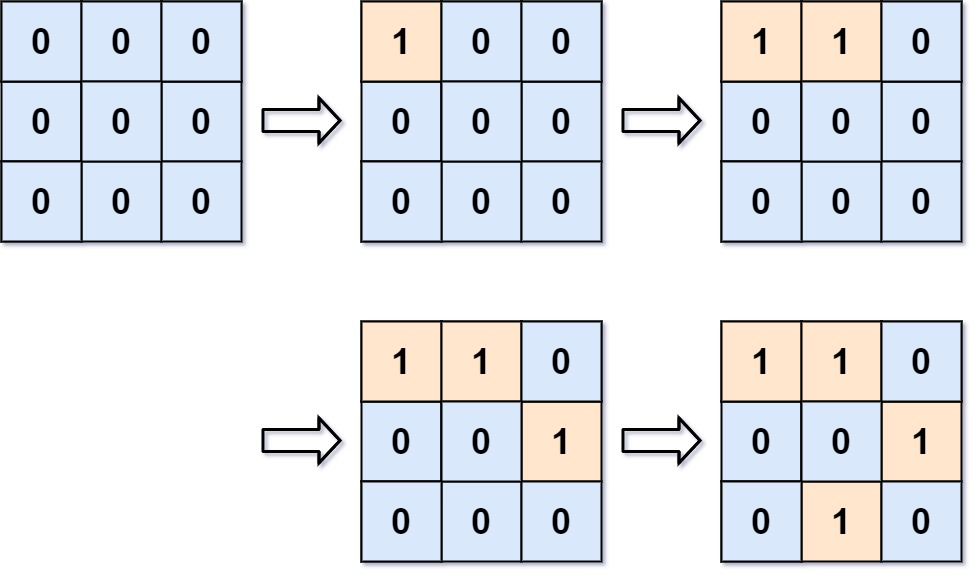### LeetCode• ㊗️
• 大家
• offer
• 多多！

## Problem

You are given an empty 2D binary grid grid of size m x n. The grid represents a map where 0’s represent water and 1’s represent land. Initially, all the cells of grid are water cells (i.e., all the cells are 0’s).

We may perform an add land operation which turns the water at position into a land. You are given an array positions where positions[i] = [ri, ci] is the position (ri, ci) at which we should operate the ith operation.

Return an array of integers answer where answer[i] is the number of islands after turning the cell (ri, ci) into a land.

An island is surrounded by water and is formed by connecting adjacent lands horizontally or vertically. You may assume all four edges of the grid are all surrounded by water.

Example 1:Input: m = 3, n = 3, positions = [[0,0],[0,1],[1,2],[2,1]]
Output: [1,1,2,3]
Explanation:
Initially, the 2d grid is filled with water.
- Operation #1: addLand(0, 0) turns the water at grid into a land. We have 1 island.
- Operation #2: addLand(0, 1) turns the water at grid into a land. We still have 1 island.
- Operation #3: addLand(1, 2) turns the water at grid into a land. We have 2 islands.
- Operation #4: addLand(2, 1) turns the water at grid into a land. We have 3 islands.


Example 2:

Input: m = 1, n = 1, positions = [[0,0]]
Output: 


## Code

class Solution {
public List<Integer> numIslands2(int m, int n, int[][] positions) {
List<Integer> result = new ArrayList<>();
if (positions == null || positions.length == 0) {
return result;
}
int[] root = new int[m * n];
Arrays.fill(root, -1);
int count = 0;
int[] xDelta = {1, -1, 0, 0};
int[] yDelta = {0, 0, 1, -1};
for (int[] position : positions) {

int x = position;
int y = position;
int index = x * n + y;
if (root[index] != -1) {    // duplicate position
continue;
}
count++;
root[index] = index;
for (int i = 0; i < 4; i++) {
int r = x + xDelta[i];
int c = y + yDelta[i];
if (isValid(m, n, r, c, root)) {
int neighborIndex = r * n + c;
int neighborRoot = findRoot(root, neighborIndex);
if (neighborRoot != index) {
root[neighborRoot] = index;
count--;
}
}
}
}
return result;
}

private boolean isValid(int m, int n, int r, int c, int[] root) {
if (r < 0 || c < 0 || r >= m || c >= n || root[r * n + c] == -1) {
return false;
}
return true;
}

private int findRoot(int[] root, int index) {
while (index != root[index]) {
root[index] = root[root[index]];
index = root[index];
}
return index;
}
}